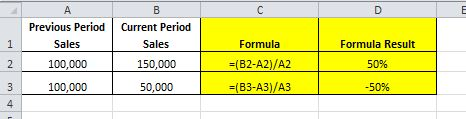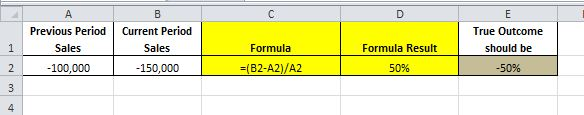# Calculate the % change

December 7, 2015

When creating reports with history, such as a weekly sales report, it is very useful to add a percentage change column against the data. It is useful for managers to see the full values but, especially when dealing with large numbers, it is more useful to interpret the change between two periods in percentage terms.

The expression for calculating a percentage change mathematically is:

% Change = (Current Period – Previous Period) / Previous Period

For example, if January sales are 10,000 and February Sales are 15,000 we would calculate:

% Change = (15,000 – 10,000) / 10,000, which equals a 50% percentage increase in sales.

This is a fairly simple concept to grasp and within Excel can be expressed like so:However, and this is usually the part that can confuse Excel users when you add negatives into the mix you can sometimes get misleading percentage changes like the following shows:Introducing the ABS Function

The ABS Function is a way to express a value as an absolute value, in other words, it ignores the sign of the value (negatives) and treats the value as a positive. This is useful in our case here because it is the combination of negative values that are causing our Excel formula to fail.

The ABS Function takes the format:

ABS(Value)

And we utilise it in this situation to make sure we only divide our difference by a positive value, this will make sure we stop getting any false negative percentages in our results. We implement like so:Our new expression:

% Change = (Current Period – Previous Period) / Absolute Value (Previous Period)

Or as expressed in Excel:

= (Current Period – Previous Period) / ABS(Previous Period)

Will work in all situations so going forward this is the way to remember how to calculate the percentage change in Excel.

Check out the comprehensive Microsoft Excel Course here.

Happy Excelling
Team Excelgoodies
www.Excelgoodies.com